# Capacitor - Types of Capacitor and Capacitance

## What is a Capacitor?

The capacitor is a device in which electrical energy can be stored. It is an arrangement of two-conductor generally carrying charges of equal magnitudes and opposite sign and separated by an insulating medium. The non-conductive region can either be an electric insulator or vacuum such as glass, paper, air or semi-conductor called as a dielectric.

Capacitor vary in shape and size, they have many important applications in electronics.

Related Physics Concepts:

### What are Capacitors Used For?

• Storing electric potential energy such as batteries.
• Filtering out unwanted frequency signals
• Delaying voltage changes when coupled with resistors.
• Used as a sensing device.
• Used in the audio system of the vehicle.
• Used to separate AC and DC.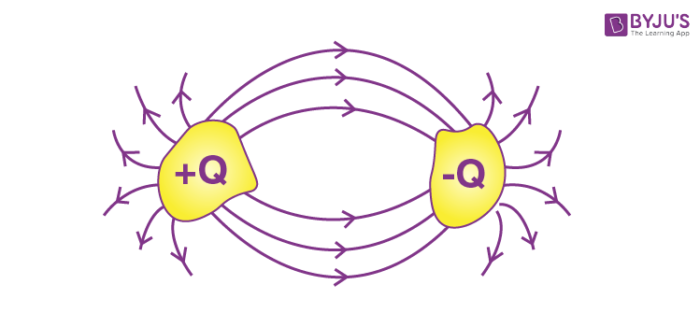One of the conductors has a positive charge +Q and it is at potential +V. whereas the other has an equal negative charge, -Q and is at potential –V.

## Charge on Capacitor

Note: Charge on the capacitor is Q.

Total charge/ the net charge on the capacitor is –Q + Q = 0.

### Circuit Symbols#### Charge Distribution Between Capacitors – Video Lesson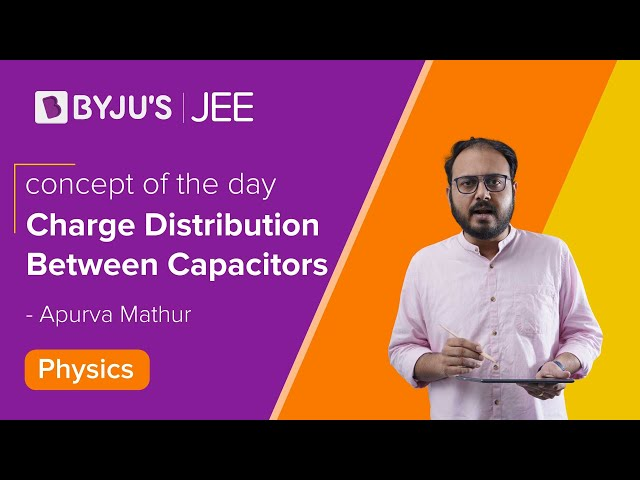## Capacitance

The charge on the capacitor (Q) is directly proportional to the potential difference (V) between the plates i.e.

$$\begin{array}{l}Q\alpha V\end{array}$$
or Q = CV

The constant of proportionality (C) is termed as the capacitance of the capacitor.

### Dimensional Formula and Unit of Capacitance

• Unit of Capacitance: Farad (F)

The capacitor value can vary from a fraction of pico-farad to more than a micro Farad. Voltage level can range from a couple to a substantial couple of hundred thousand volts.

• Dimensional Formula: M-1L-2I2T4

#### Commonly Used Scales

• $$\begin{array}{l}\mu F\end{array}$$
= 10-6F
• nF = 10-9F
• pF = 10+2F

### Factors Affecting the Capacitance

Capacitance depends on the following factor:

1. Shape and size of the conductor
2. Medium between them
3. Presence of other conductors near it.

### Calculation of Capacitance

We will try to calculate the capacitance of differently shaped capacitors, the steps are followed;

1. Assume the charge on the conductors(Q)
2. Calculate the electric field between the plates (E)
3. Calculate potential difference from electric field(V)
4. Apply the relation,
$$\begin{array}{l}C=\frac{Q}{V}\end{array}$$

## Types of Capacitor

• Parallel Plate Capacitor
• Spherical capacitor
• Cylindrical capacitor

### Parallel Plate Capacitor

The parallel plate capacitor consists of two metal plates of Area, A and is separated by a distance d. The plate on the top is given a charge +Q and that at the bottom is given the charge –Q. A potential difference of V is developed between the plates.

The separation is very small compared to the dimensions of the plate so that the effect of bending outward of electric field lines at the edges and the non-uniformity of surface charge density at the edges can be ignored.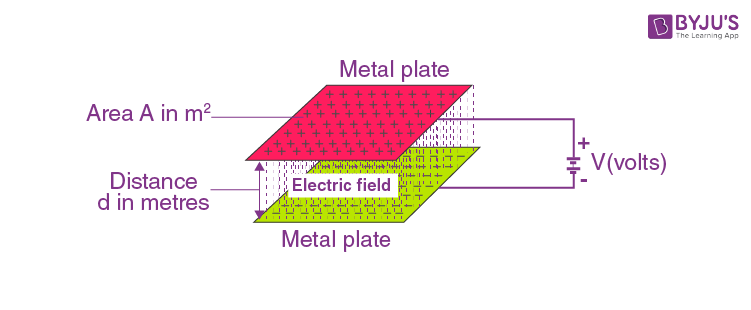The charge density on each plate of parallel plate capacitor has a magnitude of σ

σ = Q/A

From Gauss law, E = Q/ε0A

Also, E = V/d

Now taking field due to the surface charges, outside of the capacitor,

$$\begin{array}{l}E = \frac{\sigma}{2\varepsilon _0}-\frac{\sigma}{2\varepsilon _0}=0\end{array}$$

$$\begin{array}{l}Inside\;E = \frac{\sigma}{2\varepsilon _0}+\frac{\sigma}{2\varepsilon _0}=\frac{\sigma}{\varepsilon _0}=\frac{q}{A\varepsilon _0}\;\;\;\end{array}$$

$$\begin{array}{l}\frac{v}{d} = \frac{q}{A\varepsilon _0}\end{array}$$

$$\begin{array}{l}or,C = \frac{q}{v} =\frac{A\varepsilon _0}{d}\end{array}$$

This results is valid for vacuum between the capacitor plates. For other medium, then capacitance will be

$$\begin{array}{l}C = \frac{kA\varepsilon _0}{d}\end{array}$$
, where k is the dielectric constant of the medium,

$$\begin{array}{l}\varepsilon _0 = Permittivity\;of\;free\;space = 8.85\times 10^{-12}C^{2}/Nm^{2}\end{array}$$

If there is vacuum between the plates, k=1.

#### Parallel Plate Capacitor – Video Lesson### Spherical Capacitor

Let’s consider a spherical capacitor that consists of two concentric spherical shells. Suppose the radius of the inner sphere, Rin = a and radius of the outer sphere, Rout = b. The inner shell is given a positive charge +Q and the outer shell is given –Q.

The potential difference,

$$\begin{array}{l}V = \frac{q}{4\pi \epsilon _{0}ka}+\frac{-q}{4\pi \epsilon _{0}kb}\end{array}$$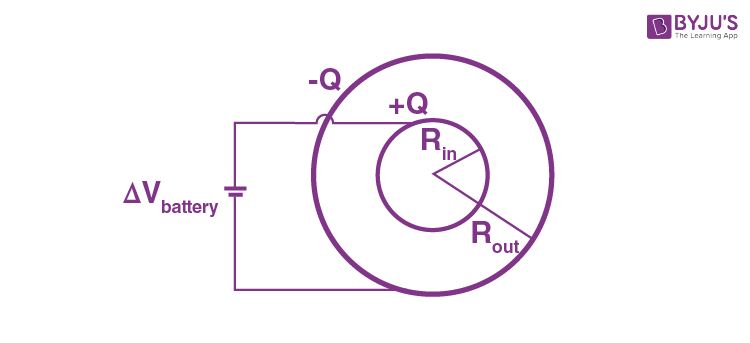$$\begin{array}{l}V = \frac{q}{4\pi \epsilon _{0}k}\left [ \frac{1}{a}-\frac{1}{b} \right ]\end{array}$$

$$\begin{array}{l}V = \frac{q}{4\pi \epsilon _{0}k}\left [ \frac{b-a}{ab} \right ]\end{array}$$

$$\begin{array}{l}C = \frac{q}{V}= \frac{q}{\frac{q}{4\pi \epsilon _{0}k}\left [ \frac{b-a}{ab} \right ]}\end{array}$$

$$\begin{array}{l}C = 4\pi \epsilon _{0}k\left [ \frac{ba}{b-a} \right ]\end{array}$$

### Cylindrical Capacitor

Consider a solid cylinder of radius, a surrounded by a cylindrical shell, b. The length of the cylinder is l and is much larger than a-b to avoid edge effects. The capacitor is charged so that the charge on the inner cylinder is +Q and the outer cylinder is –Q.

From gauss’s law,

$$\begin{array}{l}E = \frac{Q}{2\pi \varepsilon_0 rl } = \frac{\lambda}{2\pi\varepsilon _0r}\end{array}$$

Where λ = Q/l, linear charge density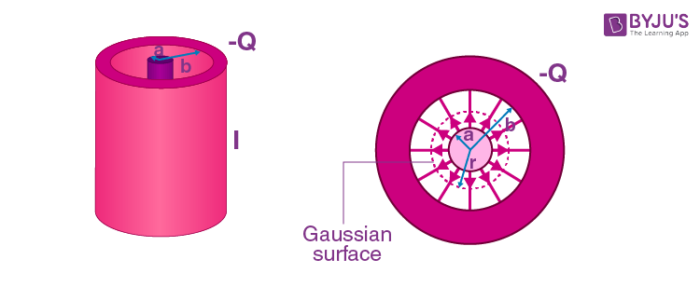The potential difference of Cylindrical Capacitor is given by,

$$\begin{array}{l}\Delta V = V_b – V_a = -\int_{a}^{b}E_rdr = -\frac{\lambda}{2\pi\varepsilon _0}\ln \left ( \frac{b}{a} \right )\end{array}$$

Where we have chosen the integration path to be along the direction of the electric field lines. As expected, the outer conductor with negative charge has a lower potential. That gives

$$\begin{array}{l}C = \frac{Q}{\left | \Delta V \right |} = \frac{\lambda L}{\lambda \ln (b/a)/2\pi\varepsilon _0} = \frac{2\pi\varepsilon _0L}{\ln(b/a)}\end{array}$$

Once again, we see that the capacitance C depends only on the geometrical L, a and b.

#### Cylindrical Capacitor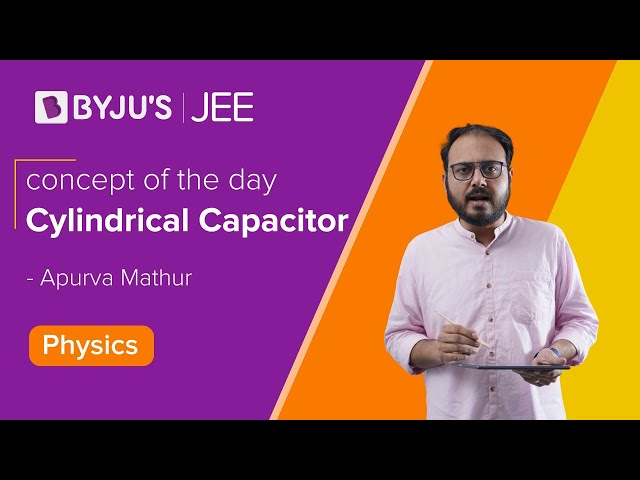## Problems on Capacitor and Capacitance

Problem 1: Find the capacitance of a conducting sphere of radius R.

Sol: Let charge Q is given to sphere. The field outside the sphere at distance r is E =

$$\begin{array}{l}=\frac{kQ}{r^{2}}\end{array}$$

$$\begin{array}{l}-\frac{dV}{dr} = E\end{array}$$

$$\begin{array}{l}\int_{0}^{v}dV = -\int_{\infty }^{R}Edr\end{array}$$

$$\begin{array}{l}V = kQ\left [ -\frac{1}{r} \right ]_{\infty }^{R}\end{array}$$

$$\begin{array}{l}V = \frac{kQ}{R}\end{array}$$

$$\begin{array}{l}C = \frac{Q}{V} = \frac{R}{1/4\pi\varepsilon _0} = 4\pi\varepsilon _0R\end{array}$$

Problem 2: A parallel plate air capacitor is made using two plates 0.2m square, spaced 1cm apart. It is connected to a 50V battery.

1. What is the capacitance?
2. What is the charge on each plate?
3. What is the electric field between two plates?
4. If the battery is disconnected and then the plates are pulled apart to a separation of 2cm, what are the answers to the above parts?

Sol:

• $$\begin{array}{l}C_{0} = \frac{\varepsilon_{0}A}{d_{0}} = \frac{8.85\times10^{-12}\times0.2\times0.2}{0.01}\end{array}$$
= 35.4 x 10-12 F
• $$\begin{array}{l}Q_0=C_{0}V_{0}=(35.4\times 10^{-12}\times50)C = 1.77\times10^{-5}C = 1770 \times 10^{-12}C\end{array}$$
• $$\begin{array}{l}E_{0} = \frac{V_{0}}{d_{0}} =\frac{50}{0.01} = 5000V/m\end{array}$$
• If the battery is disconnected, the charge on the capacitor plates remains constant while the potential difference between plates can change.

$$\begin{array}{l}C = \frac{A\varepsilon_{0}}{2d} = {1.77\times10^{-5}}\mu f\end{array}$$

$$\begin{array}{l}Q=Q_0= 1.77\times10^{-3}\mu F\end{array}$$

$$\begin{array}{l}V = \frac{Q}{C} = \frac{Q_{0}}{C_{b}/2}=2V_{0} = 100 volts\end{array}$$

$$\begin{array}{l}E = \frac{V}{C} = \frac{2V_{0}}{2d_{0}} = E_{0} = 5000 V/m\end{array}$$

Problem 3: A parallel plate conductor connected in the battery with a plate area of 3.0 cm2 and plate separation is of 3mm if the charge stored on the plate is 4.0pc. Calculate the voltage of the battery?

Sol:

Area A = 3.0 cm2 = 3.0 × 10-4 m2

$$\begin{array}{l}C_{a} = \frac{\varepsilon_{0}A}{d_{0}}\end{array}$$

$$\begin{array}{l}C_{a} = \frac{\varepsilon_{0}A}{d_{0}} = \frac{8.85\times 10^{-12}\left ( 3\times 10^{-4} \right )}{3\times 10^{-3}}\end{array}$$

C= 8.85 × 10-13

$$\begin{array}{l}C = \frac{Q}{V}\end{array}$$

$$\begin{array}{l}V = \frac{Q}{C}\end{array}$$

$$\begin{array}{l}V = \frac{4\times 10^{-12}}{8.85\times\times 10^{-13}}\end{array}$$

V = 4.52 V

## Dielectrics and Capacitance

### What are Dielectrics?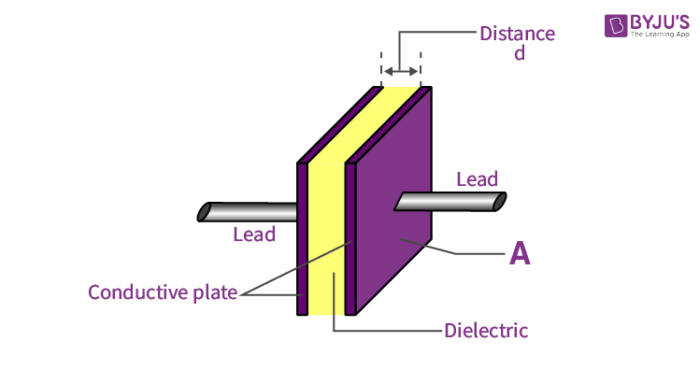It is an insulating material (non-conducting) which has no free electrons. But a microscopic displacement of charges is observed in the presence of an electric field. It is found that the capacitance increases as the space between the conducting plates are filled with dielectrics.

### Polar and Non-polar Dielectrics

Each atom is made of a positively charged nucleus surrounded by electrons. If the centre of the negatively charged electrons does not coincide with the centre of the nucleus, then a permanent dipole (separation of charges over a distance) moment is formed. Such molecules are called polar molecules. If a polar dielectric is placed in an electric field, the individual dipoles experience a torque and try to align along the field.

In non-polar molecules, the centres of the positive and negative charge distributions coincide. There is no permanent dipole moment created. But in the presence of an electric field, the centres are slightly displaced. This is called induced dipole moments.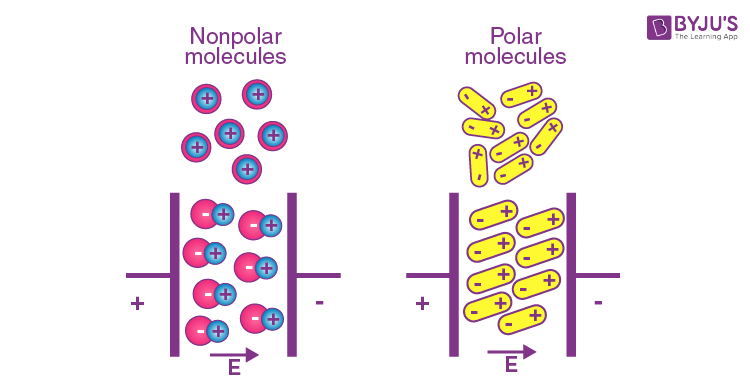### Polarization of a Dielectric Slab

It is the process of inducing charges on the dielectric and creating a dipole moment. Dipole moment appears in any volume of a dielectric. The polarization vector

$$\begin{array}{l}\overrightarrow{p}\end{array}$$
is defined as the dipole moment per unit volume.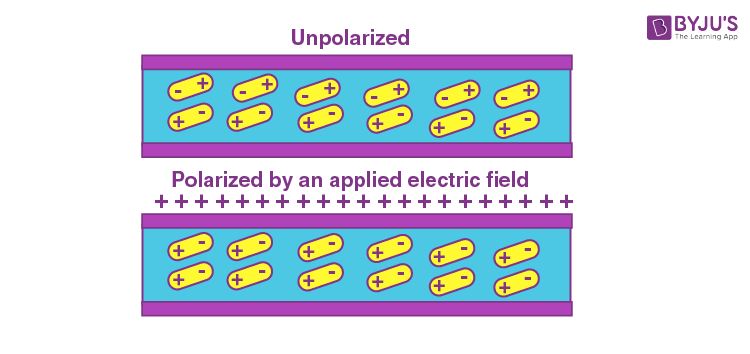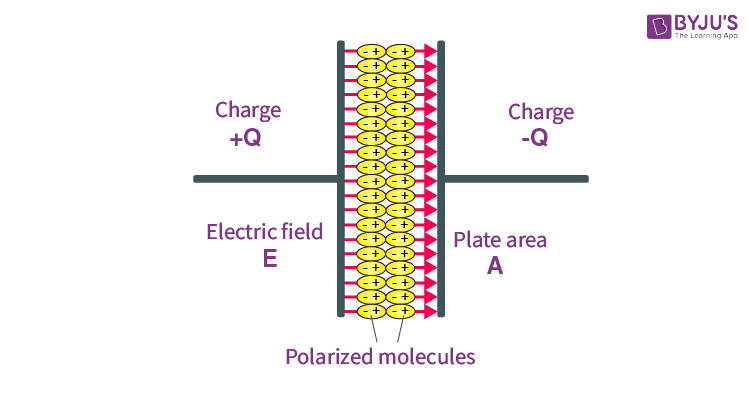## Dielectric Constant

Let

$$\begin{array}{l}\overrightarrow{{{E}_{0}}}\end{array}$$
be the electric field due to external sources and
$$\begin{array}{l}\overrightarrow{{{E}_{p}}}\end{array}$$
be the field due to polarization (induced). The resultant field is

$$\begin{array}{l}\overrightarrow{E}=\overrightarrow{{{E}_{0}}}+\overrightarrow{{{E}_{p}}}\end{array}$$
.

The induced electric field is opposite in direction to the applied field. But the resultant field is in the direction of the applied field with reduced magnitude.

$$\begin{array}{l}\overrightarrow{E}=\frac{\overrightarrow{{{E}_{0}}}}{K}\end{array}$$
K is called the dielectric constant or relative permittivity of the dielectric. For vacuum,
$$\begin{array}{l}\overrightarrow{{{E}_{p}}}\end{array}$$
= 0, K = 1. It is also denoted by ε

## Effect of Dielectric in Capacitance

### Dielectric Slabs in Series

A parallel plate capacitor contains two dielectric slabs of thickness d1, d2 and dielectric constant k1 and k2 respectively. The area of the capacitor plates and slabs is equal to A.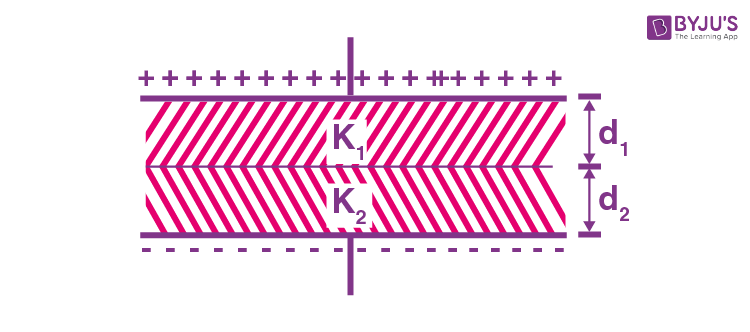Considering the capacitor as combination of two capacitors in series, the equivalent capacitance C is given by:

$$\begin{array}{l}\frac{1}{C}=\frac{1}{{{C}_{1}}}+\frac{1}{{{C}_{2}}}\end{array}$$

$$\begin{array}{l}\frac{1}{C}=\frac{{{d}_{1}}}{{{k}_{1}}\varepsilon _{0}A}+\frac{{{d}_{2}}}{{{k}_{2}}{{\varepsilon }_{0}}A}\end{array}$$

$$\begin{array}{l}C=\frac{{{\varepsilon }_{0}}A}{\frac{{{d}_{1}}}{{{k}_{1}}}+\frac{{{d}_{2}}}{{{k}_{2}}}}\end{array}$$

### Dielectric Slabs in Parallel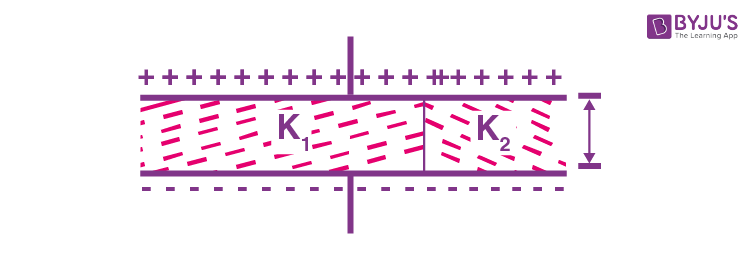Consider a capacitor with two dielectric slabs of same thickness d placed inside it as shown. The slabs have dielectric constants k1 and k2 and areas A1 and A2 respectively. Treating the combination as two capacitors in parallel,

C = C1 + C2

C =

$$\begin{array}{l}C=\frac{{{k}_{1}}{{\varepsilon }_{0}}{{A}_{1}}}{d}+\frac{{{k}_{2}}{{\varepsilon }_{0}}{{A}_{2}}}{d}\,\,\,\,\Rightarrow \,\,\,C=\frac{{{\varepsilon }_{0}}}{d}[{{k}_{1}}{{A}_{1}}+{{k}_{2}}{{A}_{2}}]\end{array}$$

## Dielectric and Vacuum

If there exits a dielectric slab of thickness t inside a capacitor whose plates are separated by distance d, the equivalent capacitance is given as:

$$\begin{array}{l}C=\frac{{{\varepsilon }_{0}}A}{\frac{t}{k}+\frac{d-t}{1}}\,\,\,\,\,\,\,\,\,\,\,\,\,\,\,\,\,\,\,(k=1\,for\,vacuum)\end{array}$$

$$\begin{array}{l}C=\frac{{{\varepsilon }_{0}}A}{\frac{t}{k}+d-t}\end{array}$$

The equivalent capacitance is not affected by changing the distance of slab from the parallel plates. If the slab is of metal, the equivalent capacitance is:

$$\begin{array}{l}C=\frac{{{\varepsilon }_{0}}A}{d-t}\end{array}$$

### Problems on Capacitance and Dielectrics

Problem 1: Three capacitors of 10μF each are connected as shown in the figure. Two of them are now filled with dielectric with K = 2, K = 2.5 as shown. Find the equivalent capacitance.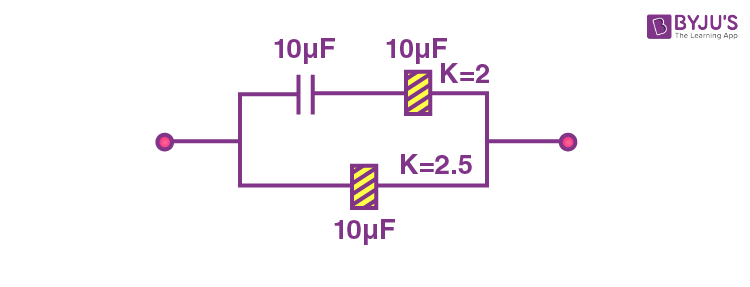After insertion of dielectrics,

C1 = 10μF; C2 = KC0 = 2 x 10 = 20μF; C3 = KC0 = 2.5 x 10 = 25 μF

$$\begin{array}{l}\,{{C}_{eff}}=\frac{10\times 20}{10+20}+25=31\frac{2}{3}\mu F\end{array}$$

Problem 2: Find the equivalent capacitance of the system shown (assume square plates).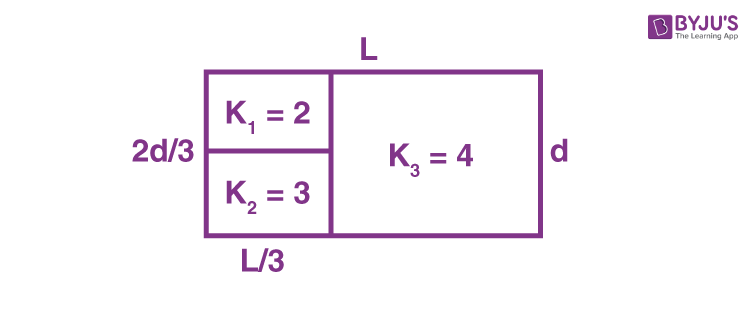Taking K1 = 2 to be series in K2 = 3

$$\begin{array}{l}\Rightarrow \,\,\frac{1}{{{c}_{left}}}=\frac{1}{\frac{(2){{\varepsilon }_{0}}\left\{ (L)\left( \frac{L}{3} \right) \right\}}{\left( \frac{d}{3} \right)}}+\frac{1}{\frac{(3){{\varepsilon }_{0}}\left\{ (L)\left( \frac{L}{3} \right) \right\}}{\left( \frac{2d}{3} \right)}}\Rightarrow \,\,\,\,{{C}_{left}}=\frac{6{{\varepsilon }_{0}}{{L}^{2}}}{7d}\end{array}$$
Now

$$\begin{array}{l}{{C}_{right}}=\frac{(4){{\varepsilon }_{0}}\left\{ (L)\left( \frac{2L}{3} \right) \right\}}{d}=\frac{8{{\varepsilon }_{0}}{{L}^{2}}}{3d}\end{array}$$

Now Cleft and Cright are in parallel

$$\begin{array}{l}\Rightarrow \,\,\,{{C}_{eq}}={{C}_{left}}+{{C}_{right}}=\frac{6{{\varepsilon }_{0}}{{L}^{2}}}{7d}+\frac{8{{\varepsilon }_{0}}{{L}^{2}}}{3d}=\frac{74{{\varepsilon }_{0}}{{L}^{2}}}{21d}\end{array}$$

Problem 3: Calculate the effective capacitance connected in series and parallel? And the capacitors connected to 40 V battery. Calculate the voltage across the capacitors for each connection type.

Given

C1 = 12 F

C2 = 6 F

Solu:

When capacitors are connected in series

$$\begin{array}{l}\frac{1}{C} = \frac{1}{C_{1}} + \frac{1}{C_{2}}\end{array}$$

$$\begin{array}{l}\frac{1}{C} = \frac{1}{12} + \frac{1}{6}\end{array}$$

$$\begin{array}{l}\frac{1}{C} = 0.25\end{array}$$

C = 4 F

$$\begin{array}{l}C = \frac{Q}{V}\end{array}$$

Q = CV

Q = 4 × 40

Q = 160 C

For the 12 F capacitor:

$$\begin{array}{l}12 = \frac{160}{V}\end{array}$$

V = 13.33V

For the 6F capacitor:

$$\begin{array}{l}6 = \frac{160}{V}\end{array}$$

V = 26.66V

When capacitors are connected in parallel

C = C1 + C2

C = 12 + 6

C = 18 F

The volatege is same as 40V across the each capacitors.

### You might also be interested in:

#### Electrostatics and Capacitor – Important Topics#### Electrostatics and Capacitor – Important Questions#### Watch this Video for More Reference## Frequently Asked Questions on Types of Capacitors and Capacitance

### Define the capacitance of a capacitor?

The capacitance of a capacitor is the ratio of the magnitude of the charge on either conductor to the potential difference between the conductors forming the capacitor.

### How can the capacitance of the parallel plate capacitor be increased?

The capacitance of the parallel plate capacitor can be increased by
Increasing the area of the plates.
Decreasing the distance between the plates.
Using a medium of higher dielectric constant.

### Does the capacitance of the capacitor change when the charge on it is doubled?

The capacitance is independent of charge. Therefore, capacitance remains the same.

### What happens to the charge on the parallel plate capacitor when the potential difference between the plates is doubled?

The charge also gets doubled.

Test your Knowledge on Capacitor Types And Capacitance